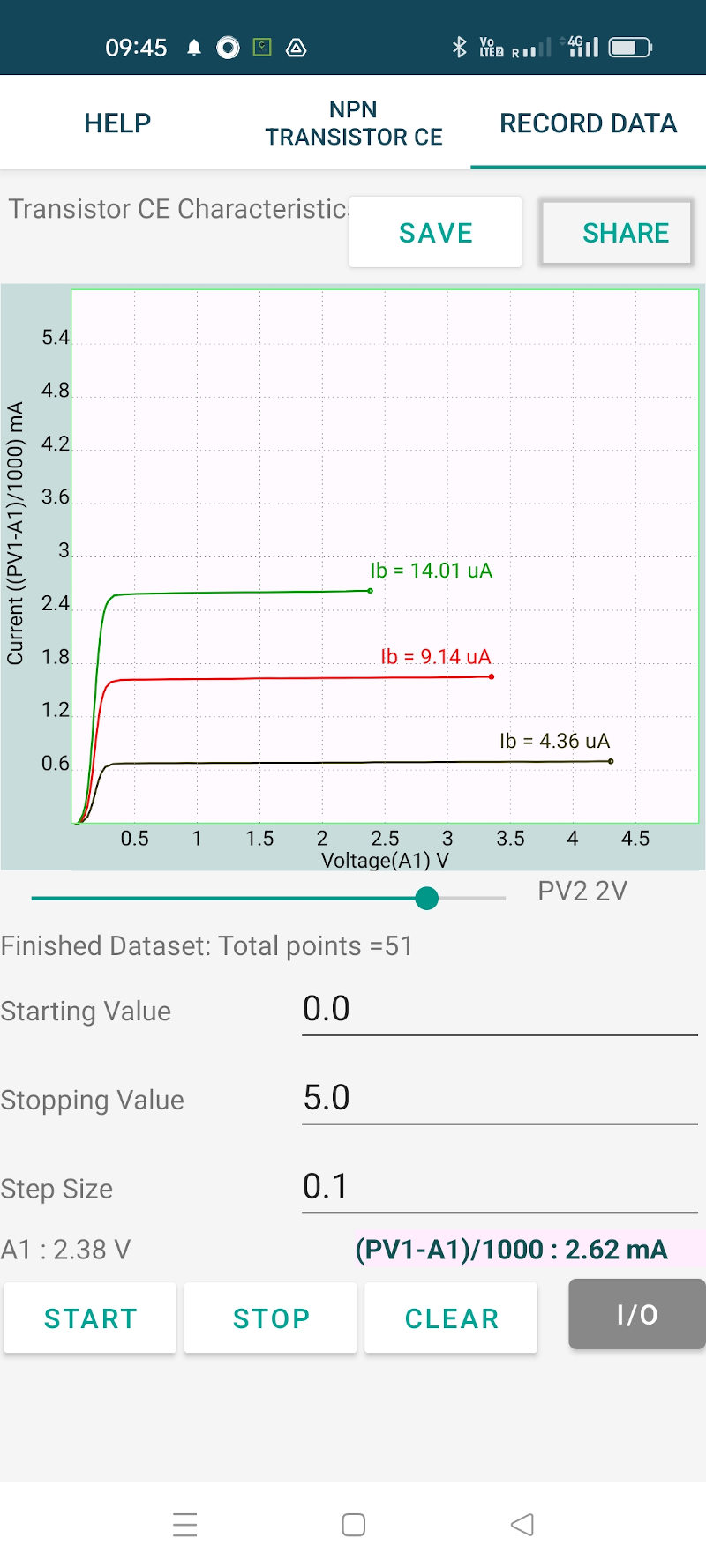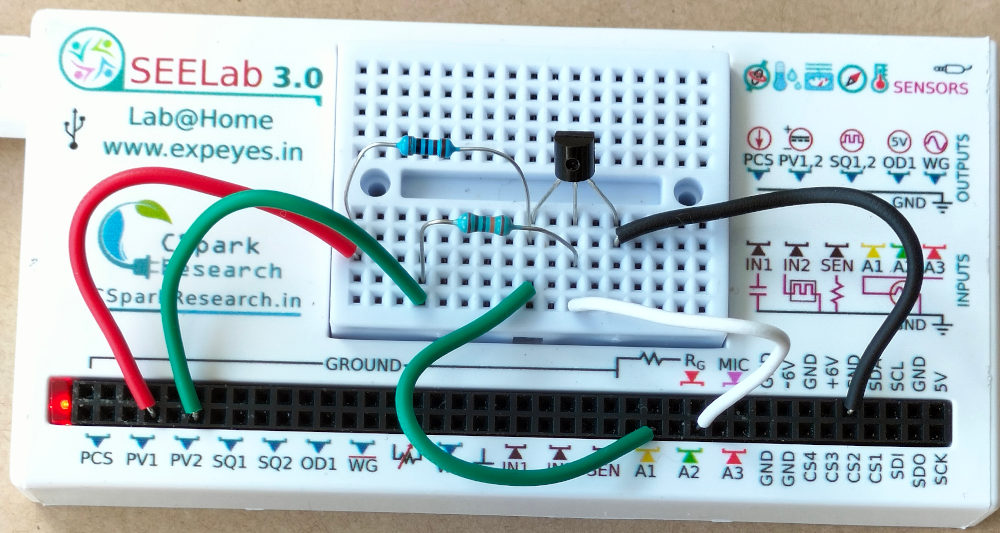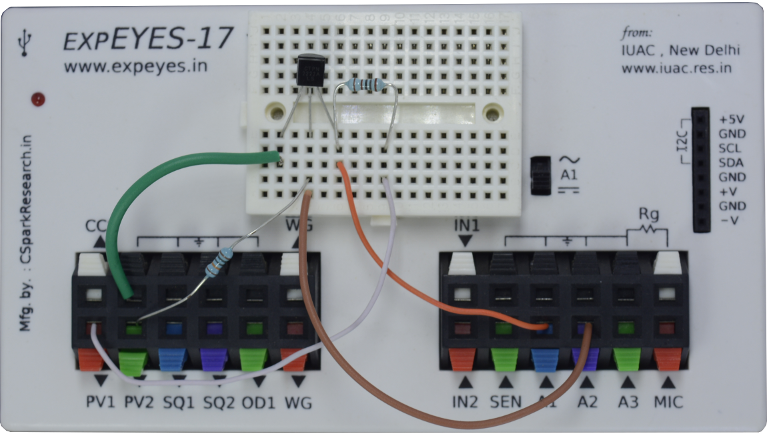# ExpEYES

## Output characteristics of an NPN transistor

The base current is set by the voltage from PV2, through a 100k resistor. The base voltage is measured to calculate the base current from Ib = (PV2-A2)/100K. The collector voltage is monitored by A1. The collector is connected to PV1, through a 1k resistor. For a selected base current, the voltage at PV1 is incremented in steps and at each step the collector voltage is measured. Corresponding collector current is calculated from i = (PV1-A1)/R. The transistor used is 2N2222, having a current gain of around 200. The experimental setup using both ExpEYES-17 and SEELab3 hardware and the results are shown below.